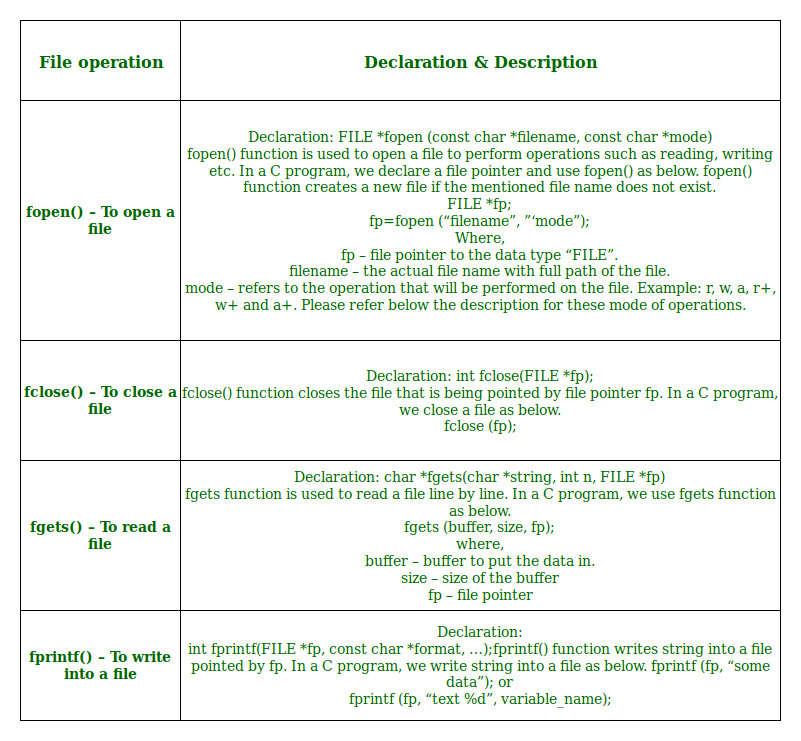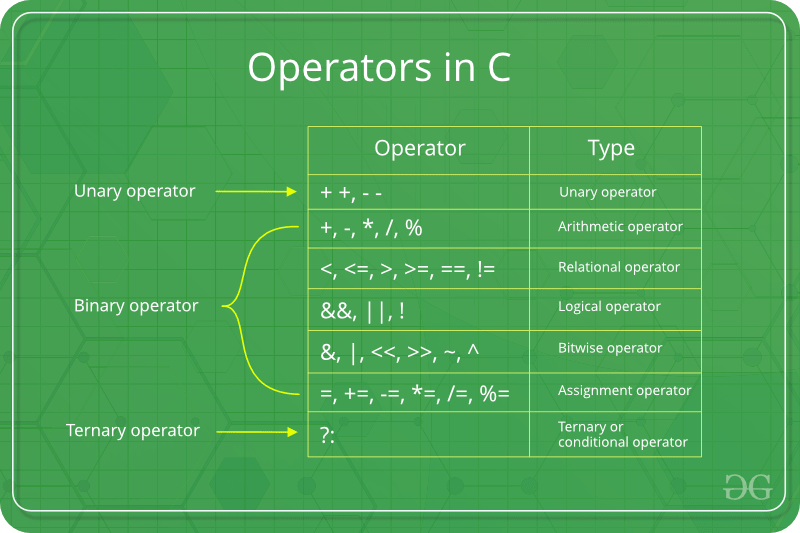# B.Tech. (SEM-I) THEORY EXAMINATION 2015-16 COMPUTER SYSTEM & PROGRAMMING IN C

Time: 3hrs
Total Marks: 100

Note:-

• There are three sections. Section A carries 20 marks, Section B carries 50 marks and Section C carries 30 marks.
• Attempt all questions. Marks are indicated against each question.
• Assume suitable data wherever necessary.

# Section – C

### Attempt any two questions from this section: (15*2 = 30)

10. What are the various types of files that can be created in C language? Also give different modes in which these files can be used wuth proper syntax. Write a program in C language to append some more text at the end of an existed text file.
File opening modes in C:

• “r” – Searches file. If the file is opened successfully fopen( ) loads it into memory and sets up a pointer which points to the first character in it. If the file cannot be opened fopen( ) returns NULL.
• “w” – Searches file. If the file exists, its contents are overwritten. If the file doesn’t exist, a new file is created. Returns NULL, if unable to open file.
• “a” – Searches file. If the file is opened successfully fopen( ) loads it into memory and sets up a pointer that points to the last character in it. If the file doesn’t exist, a new file is created. Returns NULL, if unable to open file.
• “r+” – Searches file. If is opened successfully fopen( ) loads it into memory and sets up a pointer which points to the first character in it. Returns NULL, if unable to open the file.
• “w+” – Searches file. If the file exists, its contents are overwritten. If the file doesn’t exist a new file is created. Returns NULL, if unable to open file.
• “a+” – Searches file. If the file is opened successfully fopen( ) loads it into memory and sets up a pointer which points to the last character in it. If the file doesn’t exist, a new file is created. Returns NULL, if unable to open file.

11. a) Write a C program to check whether a given square matrix is symmetric or not

 `// Simple C code for check a matrix is ` `// symmetric or not. ` ` `  `#include ` ` `  `const` `int` `MAX = 3; ` ` `  `// Fills transpose of mat[N][N] in tr[N][N] ` `void` `transpose(``int` `mat[][MAX], ``int` `tr[][MAX], ``int` `N) ` `{ ` `    ``for` `(``int` `i = 0; i < N; i++) ` `        ``for` `(``int` `j = 0; j < N; j++) ` `            ``tr[i][j] = mat[j][i]; ` `} ` ` `  `// Returns true if mat[N][N] is symmetric, else false ` `int` `isSymmetric(``int` `mat[][MAX], ``int` `N) ` `{ ` `    ``int` `tr[N][MAX]; ` `    ``transpose(mat, tr, N); ` `    ``for` `(``int` `i = 0; i < N; i++) ` `        ``for` `(``int` `j = 0; j < N; j++) ` `            ``if` `(mat[i][j] != tr[i][j]) ` `                ``return` `0; ` `    ``return` `1; ` `} ` ` `  `// Driver code ` `int` `main() ` `{ ` `    ``int` `mat = { { 1, 3, 5 }, ` `                      ``{ 3, 2, 4 }, ` `                      ``{ 5, 4, 1 } }; ` ` `  `    ``if` `(isSymmetric(mat, 3) == 1) ` `        ``printf``(``"Yes"``); ` `    ``else` `        ``printf``(``"No"``); ` `    ``return` `0; ` `} `

11. (b) Define data types in C. Discuss primitive data types in terms of memory size, format specifier and range.

Each variable in C has an associated data type. Each data type requires different amounts of memory and has some specific operations which can be performed over it. Let us briefly describe them one by one:

Following are the examples of some very common data types used in C:

• char: The most basic data type in C. It stores a single character and requires a single byte of memory in almost all compilers.
• int: As the name suggests, an int variable is used to store an integer.
• float: It is used to store decimal numbers (numbers with floating point value) with single precision.
• double: It is used to store decimal numbers (numbers with floating point value) with double precision.

Different data types also have different ranges upto which they can store numbers. These ranges may vary from compiler to compiler. Below is list of ranges along with the memory requirement and format specifiers on 32 bit gcc compiler.

Data Type Memory (bytes) Range Format Specifier
short int 2 -32, 768 to 32, 767 %hd
unsigned short int 2 0 to 65, 535 %hu
unsigned int 4 0 to 4, 294, 967, 295 %u
int 4 -2, 147, 483, 648 to 2, 147, 483, 647 %d
long int 4 -2, 147, 483, 648 to 2, 147, 483, 647 %ld
unsigned long int 4 0 to 4, 294, 967, 295 %lu
long long int 8 -(2^63) to (2^63)-1 %lld
unsigned long long int 8 0 to 18, 446, 744, 073, 709, 551, 615 %llu
signed char 1 -128 to 127 %c
unsigned char 1 0 to 255 %c
float 4 %f
double 8 %lf
long double 12 %Lf

We can use the sizeof() operator to check the size of a variable.

11. (c) List out various file operations in ‘C’. Write a C program to count the number of characters in a file.
So far the operations using C program are done on a prompt / terminal which are not stored anywhere. But in software industry, most of the programs are written to store the information fetched from the program. One such way is to store the fetched information in a file. Different operations that can be performed on a file are:

1. Creation of a new file (fopen with attributes as “a” or “a+” or “w” or “w++”)
2. Opening an existing file (fopen)
3. Reading from file (fscanf or fgetc)
4. Writing to a file (filePointerrintf or filePointeruts)
5. Moving to a specific location in a file (fseek, rewind)
6. Closing a file (fclose)

The text in the brackets denotes the functions used for performing those operations.

Functions in File Operations:12. (a) Write the difference between type conversion and type casting. What are the escape sequences characters?
In C programming language, there are 256 numbers of characters in character set. The entire character set is divided into 2 parts i.e. the ASCII characters set and the extended ASCII characters set. But apart from that, some other characters are also there which are not the part of any characters set, known as ESCAPE characters.

List of Escape Sequences

```\a    Alarm or Beep
\b    Backspace
\f    Form Feed
\n    New Line
\r    Carriage Return
\t    Tab (Horizontal)
\v    Vertical Tab
\\    Backslash
\'    Single Quote
\"    Double Quote
\?    Question Mark
\ooo  octal number
\0    Null```

Example:

 `// C program to illustrate ` `// \b escape sequence ` `#include ` `int` `main(``void``) ` `{ ` `    ``// \b - backspace character transfers ` `    ``// the cursor one character back with ` `    ``// or without deleting on different ` `    ``// compilers. ` `    ``printf``(``"Hello Geeks\b\b\b\bF"``); ` `    ``return` `(0); ` `} `

Output:

```The output is dependent upon compiler.
```

12. (b) What are the different types of operators in C language and also write down the difference between the associativity and precedence of operators.Operators are the foundation of any programming language. Thus the functionality of C/C++ programming language is incomplete without the use of operators. We can define operators as symbols that helps us to perform specific mathematical and logical computations on operands. In other words we can say that an operator operates the operands.
For example, consider the below statement:

```c = a + b;
```

Here, ‘+’ is the operator known as addition operator and ‘a’ and ‘b’ are operands. The addition operator tells the compiler to add both of the operands ‘a’ and ‘b’. C/C++ has many built-in operator types and they can be classified as:

• Arithmetic Operators: These are the operators used to perform arithmetic/mathematical operations on operands. Examples: (+, -, *, /, %, ++, –).
• Relational Operators: Relational operators are used for comparison of the values of two operands. For example: checking if one operand is equal to the other operand or not, an operand is greater than the other operand or not etc. Some of the relational operators are (==, >=, <= ). To learn about each of these operators in details go to this link.
• Logical Operators:  Logical Operators are used to combine two or more conditions/constraints or to complement the evaluation of the original condition in consideration. The result of the operation of a logical operator is a boolean value either true or false. To learn about different logical operators in details please visit this link.
• Bitwise Operators: The Bitwise operators is used to perform bit-level operations on the operands. The operators are first converted to bit-level and then calculation is performed on the operands. The mathematical operations such as addition, subtraction, multiplication etc. can be performed at bit-level for faster processing. To learn bitwise operators in details, visit this link.
• Assignment Operators: Assignment operators are used to assign value to a variable. The left side operand of the assignment operator is a variable and right side operand of the assignment operator is a value. The value on the right side must be of the same data-type of variable on the left side otherwise the compiler will raise an error.
• Other Operators: Apart from the above operators there are some other operators available in C or C++ used to perform some specific task. Some of them are discussed here:
• sizeof operator: sizeof is a much used in the C/C++ programming language. It is a compile time unary operator which can be used to compute the size of its operand. The result of sizeof is of unsigned integral type which is usually denoted by size_t. Basically, sizeof operator is used to compute the size of the variable. To learn about sizeof operator in details you may visit this link.
• Comma Operator: The comma operator (represented by the token, ) is a binary operator that evaluates its first operand and discards the result, it then evaluates the second operand and returns this value (and type). The comma operator has the lowest precedence of any C operator. Comma acts as both operator and separator. To learn about comma in details visit this link.
• Conditional Operator: Conditional operator is of the form Expression1 ? Expression2 : Expression3 . Here, Expression1 is the condition to be evaluated. If the condition(Expression1) is True then we will execute and return the result of Expression2 otherwise if the condition(Expression1) is false then we will execute and return the result of Expression3. We may replace the use of if..else statements by conditional operators. To learn about conditional operators in details, visit this link.

My Personal Notes arrow_drop_upCheck out this Author's contributed articles.

If you like GeeksforGeeks and would like to contribute, you can also write an article using contribute.geeksforgeeks.org or mail your article to contribute@geeksforgeeks.org. See your article appearing on the GeeksforGeeks main page and help other Geeks.

Please Improve this article if you find anything incorrect by clicking on the "Improve Article" button below.

Article Tags :

Be the First to upvote.

Please write to us at contribute@geeksforgeeks.org to report any issue with the above content.